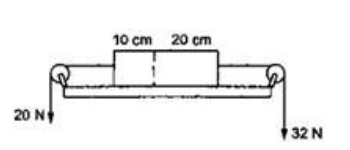Deepak Scored 45->99%ile with Bounce Back Crack Course. You can do it too!

# Solve the following :

Question:

Figure shows a uniform rod of length $30 \mathrm{~cm}$ having a mass of $3.0 \mathrm{~kg}$. The strings shown in the figure are pulled by constant forces of $20 \mathrm{~N}$ and $32 \mathrm{~N}$. Find the force exerted by the $20 \mathrm{~cm}$ part of the rod on the $10 \mathrm{~cm}$ part. All the surfaces are smooth and the strings and the pulleys are light.Solution:

$\frac{m}{l}=\frac{3}{30 \times 10^{-2}}=10 \mathrm{~kg} / \mathrm{m}$

For $0.1 \mathrm{~m}->m_{A}=10 \times 0.10=1 \mathrm{~kg}$

For $0.2 \mathrm{~m}->\mathrm{m}_{\mathrm{B}}=10 \times 0.20=2 \mathrm{~kg}$

$R-20=1 a$

$a+20=32-2 a=>3 a=12$

$\Rightarrow a=4 \mathrm{~m} / \mathrm{s}^{2} \ldots$  $\{$ a is towards right as $32 N>20 N\}$

$\mathrm{R}=20+1 a=24 \mathrm{~N}$